[Top] [Next] [Prev]

## B.1 Basic Electricity

In this subsection we introduce the basic terminology, fundamental quantities, and laws of electricity.

### B.1.1 Terminology

Electricity is energy that can be transported. An electric circuit consists of an energy source, such as a battery or power supply, and interconnected electrical components implementing a useful function. The connections are formed by wires, also known as conductors, which are made of materials such as copper or some other metal that can conduct electricity. Electrical charge transported across a conductor is called electric current. Charge is carried by electrons, which are negatively charged, or by positively charged ions in the conductor. Current is the intensity of the flow of charge. Between two points in a circuit, electrons flow from the more negatively charged point toward the one that is more positively charged. Positive charges, sometimes called holes, move in the opposite direction. By convention, current flows in the direction of holes, which is opposite to the direction of electron flow.

Voltage is associated with any two points in the circuit, and represents the difference in electrical potential between those points. Stated differently, voltage is the electric force that causes electrons to flow in a circuit. Voltage is defined as a relative quantity. In a typical digital circuit, the lowest possible voltage is called ground and is arbitrarily assigned 0 volts. In most existing digital circuits, the highest possible voltage value is defined not to exceed 5.5 volts `(`industry is starting to move toward a 3.3-volt power supply`)`. In digital systems, we assign logic 1 to "high" voltages and 0 to "low" voltages, but these assignments are somewhat arbitrary. For TTL technology of the kinds described in this book, a voltage in the range of 0 to 0.4 volts is interpreted as logic 0, while 2.5 to 5.5 volts is interpreted as a logic 1. Voltages outside these ranges are not guaranteed to be interpreted as either a 0 or a 1.

The fundamental concepts of electricity can often be described by analogy with water. The greater the electrical potential, the larger the voltage, and the greater the force on the flow of the charge-carrying electrons. Think of a waterfall. A large voltage corresponds to a waterfall of great height. As a water molecule flows "downhill," a good deal of pressure is exerted on it by gravity and the force of water behind it. By analogy, water molecules correspond to electrons and electrical current corresponds to the speed of the water flow.

Suppose that the voltage difference is 0, so that both points in the circuit are at the same potential. In this case, the water is a stagnant pool, with no water flow, and there is no current. Given a waterfall of only modest height, the water trickles slowly downhill. This is analogous to a small current. But if the waterfall is of a great height, the flow of water will be forceful and the current is large.

Whereas conductors transport electricity, other materials, called insulators, are impervious to electricity. An important class of materials is the semiconductors, materials that can change from being conductors at one moment to being insulators at the next. This makes it possible to form electrically controlled switches, which are at the heart of all digital logic circuits.

### B.1.2 Fundamental Quantities and Laws

The key electrical quantities and their units are shown in Figure B.1.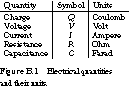Charge measures the number of positive or negative charges at a given point in the circuit and is described in units of coulombs. One coulomb is equivalent to the charge on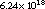electrons. Voltage is the difference in electrical potential between two points in a circuit and is measured in volts. At a given point in a circuit, current is the change in charge as a function of time. Thus, one ampere is defined to be one coulomb per second.

Resistance Figure B.1 introduces two important new quantities: resistance and capacitance. Resistance is the "friction" that limits current. Doubling resistance cuts the current in half. When two resistors are connected in series, one immediately following the other, their resistances add. Placing two resistors in parallel results in a resistance that is less than the component resistances. To be more precise, if the resistances, measured in ohms, are R1 and R2, the parallel resistance will be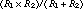.

A short circuit is a path of conductors with no `(`or very low`)` resistance. An open circuit is a conductive path with infinite resistance. Semiconductor materials make it possible to construct connections between two points that can be varied between low and high resistance.

One of the most important expressions for analyzing electrical circuits is Ohm's law `(`for the German scientist Georg Simon Ohm`)`. It describes the relationship between voltage `(`V`)`, current `(`I`)`, and resistance `(`R`)` as follows:To understand this relationship, let's consider the water analogy again. V is the height of the waterfall. We can think of R as inversely proportional to the diameter of a water pipe: a high resistance corresponds to a narrow pipe, a low resistance to a wide pipe. A narrow pipe restricts the flow more than a wide pipe. With a high resistance, current is reduced, because fewer electrons can move through the conductor per unit time. By reducing the resistance `(`that is, increasing the cross section of the pipe`)`, we increase the flow of electrons.

An alternative formulation of Ohm's law allows us to describe resistance as a function of voltage and current: R `=` V/I. Thus, if a power supply provides voltage V and the current is measured as I, then the resistance of the circuit being driven by the power supply is R.

Capacitance Capacitance is the ability to store charge and is measured in units of farads `(`named for the great 19th-century British scientist Michael Faraday`)`. A capacitor is a device with two parallel conducting plates separated by a nonconducting material. Placing negative charges on one plate will attract positive charges to the other plate. A capacitor uses current to charge the plates up slowly to a new voltage. Once charge is stored, the capacitor can also provide a "discharge" current to the rest of the circuit. Thus, capacitors are often used to smooth out variations in the current provided by the circuit's power supply.

Continuing with our water analogy, a capacitor behaves much like a water holding tank. A hole at the bottom of the tank provides a steady "outflow" of current, even though the inflow may be sporadic.

Charge, voltage, and capacitance are related by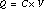Charge is equal to capacitance times voltage. By placing a voltage V across a capacitor of C farads, we can store a charge of Q coulombs.

RC Delay There is an interesting relationship between time, resistance, and capacitance. Consider how long it takes to charge up a discharged capacitor.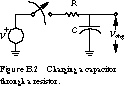Figure B.2 shows a possible setup. In the schematic, the voltage source is labeled V, the resistor R, and the capacitor C.

We assume that the capacitor is completely discharged and the switch is in the open position. When the switch is closed, the power supply begins to charge up the capacitor toward the voltage Vchg. If you measure the voltage across the capacitor with a voltmeter, initially the voltage changes very quickly, but then it slows down.

There is a precise relationship between the resistance and capacity of the circuit and the time it takes to charge the capacitor. It is directly related to, also known as the RC time constant `(`it may seem strange that ohms times farads is seconds, but this is the case`)`. After one RC delay, the capacitor is charged up to slightly more than 60% of its final value. After two RC delays, it reaches almost 90% of its final value. It takes five RC delays before the capacitor reaches 99% of its final value. This is shown in Figure B.3.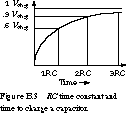RC delays play an important role in determining the true performance of digital circuits. Even though wires are excellent conductors, they do present some resistance to the current flow. But even more important, wires introduce capacitance: a wire forms one plate of a capacitor whose second plate is the circuit board itself. Changes in voltages on wires require this capacitance to be either charged or discharged, and this translates into a significant source of delay in real circuits.

[Top] [Next] [Prev]